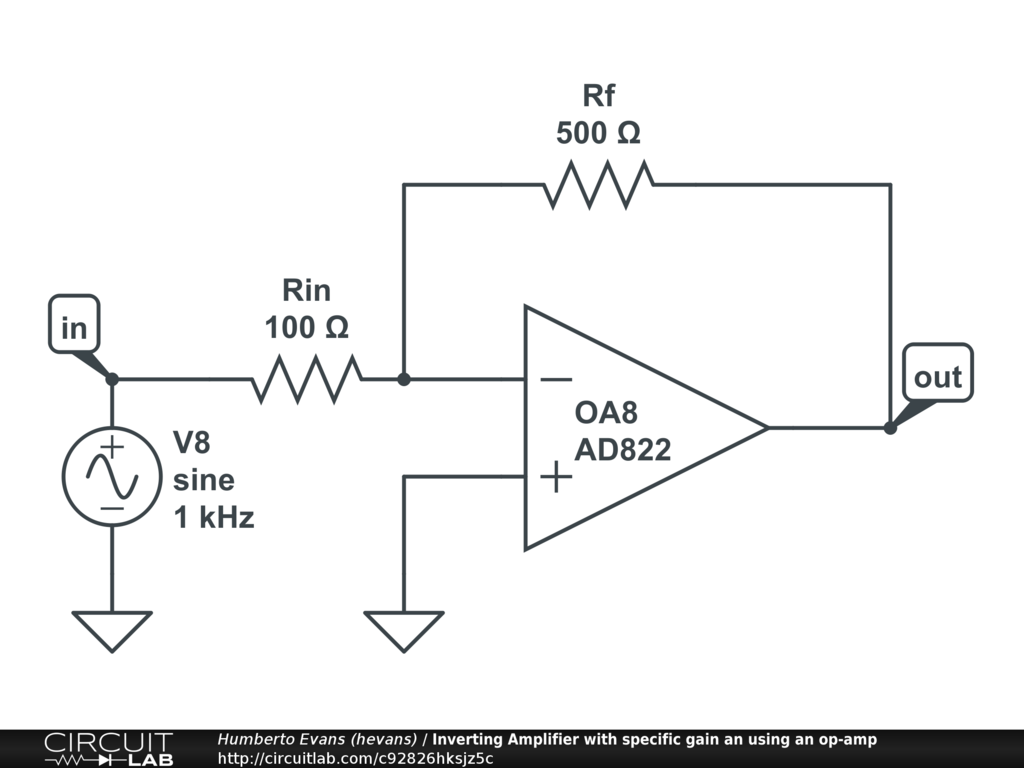## Inverting Amplifier: How to build and simulate op-amp circuit with a specific gain

#### Jun 29 2020, 12:00 PM PDT · 0 comments »

In this tutorial video we build and simulate in an inverting amplifier with a chosen gain using an op-amp and other passive elements. We go over a few key concepts of an op-amps, introduce negative feedback, and build out a circuit that allows you to choose a specific gain for your inverting amplifier.

### Circuit### Transcript:

So I'm going to open up my CircuitLab editor here, and find the operational amplifier section, and I'm gonna drop this op amp onto my circuit. Op amps have lots of super cool properties, but I'm going to be using two big ones.

First, an op amp takes the difference of the two input voltages, and multiples that difference by a really really high number at the output voltage. This multiplication factor is the open loop gain of an op amp. We can see it here in the properties, it's the A_OL number, notice how all the device models have a different number, but the important thing is that number is a super high number, look em they're all in the hundreds of thousands or millions.

The other property is that no current (in reality very very little current) flows into or out of either of the input terminals. This will be important later.

The opamp models CircuitLab model the real devices based on the numbers in their datasheets. An "ideal" op amp which you may run into a problem set just has these properties maximally true, so the open loop gain is infinite and the input currents are actually 0.

Lets see these two properties in our simulation. [Show open loop gain in CircuitLab editor]

Ok so that's neat but not that useful because I want to be able to control this gain, just having an arbitrarily large number out here isn't helpful. So the first neat trick I'm going to use is negative feedback.

I'm gonna grab the output terminal and let's reason about what happens when I connect it directly to the minus input terminal. In this configuration, if something like a bit of noise in the universe was to make this voltage go up at the non inverting terminal, the output goes up, and the inverting input goes up, which makes the output go down. That output going down is fighting the original change.. And it balances out when there’s no difference between the two input terminals.

In this configuration, with negative feedback the op amp works to subtract any difference between the two input terminals. Meaning the inverting terminal will follow the non inverting terminal. We made a follower circuit!

So we are not there yet, let's introduce one more thing. I'm going to add a voltage source here, and two resistors. And I'm gonna call this one Rf, and this one Rin. Lets reason about this circuit. What is our output? We know in this configuration the difference between these two terminals is 0. The only way it can do that is by changing its output voltage and then measuring the two inputs again. We also know that no current can flow into or out of our input terminals. So I put a signal voltage here of 1, and if the op amp is forcing this to be 0, then our trusty friend V=IR says there is current flowing through this resistor, the other rule of the op amp says that current can't go into this terminal, so it has to go through this other resistor Rf. Again our trusty friend says if there is an IR there has to be voltage drop, and since it's the same I and R I think this voltage at out2 is going to be -1, and by golly it is.

So what if I make this Rf twice as large. Same input V, same Rin, so this current is the same, but now r is twice as much. Again V=IR so twice as much R and same I means twice as much Vout. Is it? Yes it is bloody exciting.

This means that just by changing the ratio of these resistors I can manipulate this circuit to have an arbitrary gain at its output!

You can find a link to this Circuit in the description of this video, you can open it up, change it and simulate it yourself to see what happens. If you found this helpful please like the video, and we will see you next time.